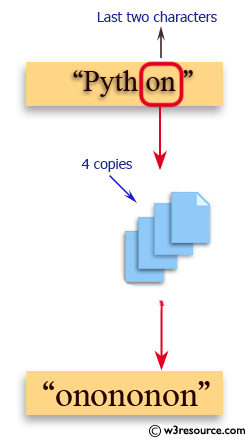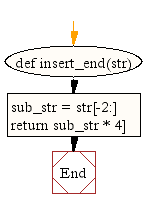﻿ Python: Get a string made of 4 copies of the last two characters of a specified string - w3resource# Python: Get a string made of 4 copies of the last two characters of a specified string

## Python String: Exercise-17 with Solution

Write a Python function to get a string made of 4 copies of the last two characters of a specified string (length must be at least 2).Sample Solution:-

Python Code:

``````def insert_end(str):
sub_str = str[-2:]
return sub_str * 4

print(insert_end('Python'))
print(insert_end('Exercises'))
```
```

Sample Output:

```onononon
eseseses
```

Flowchart:## Visualize Python code execution:

The following tool visualize what the computer is doing step-by-step as it executes the said program:

Python Code Editor:

Have another way to solve this solution? Contribute your code (and comments) through Disqus.

What is the difficulty level of this exercise?

Test your Programming skills with w3resource's quiz.

﻿

## Python: Tips of the Day

Performs right-to-left function composition:

Example:

```from functools import reduce

def tips_compose(*fns):
return reduce(lambda f, g: lambda *args: f(g(*args)), fns)
add6 = lambda x: x + 6
multiply = lambda x, y: x * y
```36